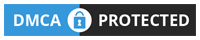# Siemens S7-1200 “Modbus RTU” FUJI Frenic VFD Tutorial

Hello everyone, today plc247.com would like to continue to share with you Series using PLC Siemens S7-1200 to control various devices. Today we will control the FUJI Frenic Series inverter

FUJI Frenic inverters are very diverse in types including: Mini, Eco, Multi, Mega
And today I use FUJI Frenic Mini to make this project

### Connection Diagram

Using module S7-1200 combined with CB1241

#### FUJI FRENIC VFD Communication Configuration

In addition to the basic parameters of the Motor, we need to set the communication parameters as follows:

+ H30 = 3 (Run & Frequency Command via RS 485)

+ Y01 = 1 (Slave Station No.)

+ Y02 = 0

+ Y03 = 2.0 ( Timer Error Detect)

+ Y04 = 2 (9600bps)

+ Y05 = 0 (8bit)

+ Y06 = 1 (Even)

+ Y07 = 1 (1bit)

+ Y10 = 0 (Modbus-RTU)

+ Y99 = 0 (Folow H30 Funtion)

This is the layout table of the register areas corresponding to the Hexa address of the inverter

##### The registers receive control commands from the PLC

>>> Frequency Reference = S (07hex) + 05 = 705 (hex) = 1797 (dec)
PLC Frequency Set Address = 40001 + 1797 = 41798 (dec)

>>> Operation Command = S (07hex) + 06 = 706 (hex) = 1798 (dec)
PLC Motor Control Address = 40001 + 1798 = 41799 (dec)

• Set 41799 = 1 >>> Run Forward
• Set 41799 = 2 >>> Run Revese
• Set 41799 = 0 >>> Stop
##### Data Feedback Registers

In this project I only read 2 Data included:

+ Output Frequency = M (08hex) + 09 = 809 (hex) = 2057 (dec)
>>> PLC Address = 40001 + 2057 = 42058 (Dec)

+ Output Voltage = M (08hex) + 12 = 80C (hex) = 2060 (dec)
>>> PLC Address = 40001 + 2060 = 42061 (dec)

#### Siemens S7-1200 Communication Configuration

S7 1200 CB1241 Configurator

Creating a Data Block for Modbus

### PLC Programming

+ Frequency Setting

+ Motor Control Run

### Video Tutorial

======

Documentation and Software:

So plc247.com has finished guiding you to connect PLC Siemens and FUJI Frenic Mini inverter. If you have any questions, please comment below the article, I will answer you as soon as possible.

Best Regards!#### plc247.com

•Patrícia Pesti says:
•plc247.com says: